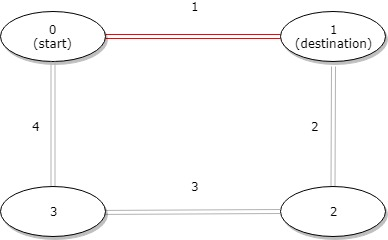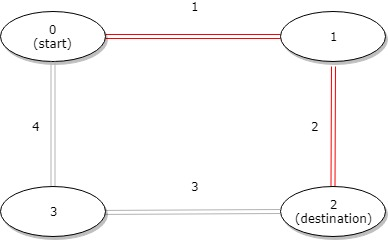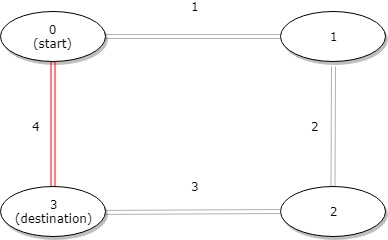##### Welcome to Subscribe On Youtube

Formatted question description: https://leetcode.ca/all/1184.html

# 1184. Distance Between Bus Stops (Easy)

A bus has n stops numbered from 0 to n - 1 that form a circle. We know the distance between all pairs of neighboring stops where distance[i] is the distance between the stops number i and (i + 1) % n.

The bus goes along both directions i.e. clockwise and counterclockwise.

Return the shortest distance between the given start and destination stops.

Example 1:Input: distance = [1,2,3,4], start = 0, destination = 1
Output: 1
Explanation: Distance between 0 and 1 is 1 or 9, minimum is 1.

Example 2:Input: distance = [1,2,3,4], start = 0, destination = 2
Output: 3
Explanation: Distance between 0 and 2 is 3 or 7, minimum is 3.


Example 3:Input: distance = [1,2,3,4], start = 0, destination = 3
Output: 4
Explanation: Distance between 0 and 3 is 6 or 4, minimum is 4.


Constraints:

• 1 <= n <= 10^4
• distance.length == n
• 0 <= start, destination < n
• 0 <= distance[i] <= 10^4

Companies:

Related Topics:
Array

## Solution 1.

• class Solution {
public int distanceBetweenBusStops(int[] distance, int start, int destination) {
int totalDistance = 0;
for (int num : distance)
totalDistance += num;
int min = Math.min(start, destination), max = Math.max(start, destination);
int distance1 = 0;
for (int i = min; i < max; i++)
distance1 += distance[i];
int distance2 = totalDistance - distance1;
return Math.min(distance1, distance2);
}
}

############

class Solution {
public int distanceBetweenBusStops(int[] distance, int start, int destination) {
if (start > destination) {
return distanceBetweenBusStops(distance, destination, start);
}
int a = 0, b = 0;
for (int i = 0; i < distance.length; ++i) {
if (i >= start && i < destination) {
a += distance[i];
} else {
b += distance[i];
}
}
return Math.min(a, b);
}
}

• // OJ: https://leetcode.com/problems/distance-between-bus-stops/
// Time: O(N)
// Space: O(1)
class Solution {
public:
int distanceBetweenBusStops(vector<int>& distance, int start, int destination) {
if (start == destination) return 0;
if (start > destination) swap(start, destination);
int sum = 0, path = 0, N = distance.size();
for (int i = 0; i < N; ++i) {
if (i >= start & i < destination) path += distance[i];
sum += distance[i];
}
return min(path, sum - path);
}
};

• class Solution:
def distanceBetweenBusStops(
self, distance: List[int], start: int, destination: int
) -> int:
if start > destination:
start, destination = destination, start
a = sum(distance[start:destination])
b = sum(distance[:start]) + sum(distance[destination:])
return min(a, b)

############

# 1184. Distance Between Bus Stops
# https://leetcode.com/problems/distance-between-bus-stops/

class Solution:
def distanceBetweenBusStops(self, distance: List[int], start: int, end: int) -> int:
a = min(start, end)
b = max(start, end)

return min(sum(distance[a:b]), sum(distance) - sum(distance[a:b]))


• func distanceBetweenBusStops(distance []int, start int, destination int) int {
if start > destination {
return distanceBetweenBusStops(distance, destination, start)
}
a, b := 0, 0
for i, v := range distance {
if i >= start && i < destination {
a += v
} else {
b += v
}
}
if a < b {
return a
}
return b
}

• /**
* @param {number[]} distance
* @param {number} start
* @param {number} destination
* @return {number}
*/
var distanceBetweenBusStops = function (distance, start, destination) {
if (start > destination) {
return distanceBetweenBusStops(distance, destination, start);
}
let a = 0;
let b = 0;
for (let i = 0; i < distance.length; ++i) {
if (i >= start && i < destination) {
a += distance[i];
} else {
b += distance[i];
}
}
return Math.min(a, b);
};


• function distanceBetweenBusStops(
distance: number[],
start: number,
destination: number,
): number {
const s = distance.reduce((a, b) => a + b, 0);
let a = 0;
const n = distance.length;
while (start != destination) {
a += distance[start];
start = (start + 1) % n;
}
return Math.min(a, s - a);
}